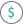#PV (PQL - xl)

This function returns the present value of an investment based on the interest rate, number and amount of periodic payments, and future value. The present value is the total amount that a series of future payments is worth now

• Library: PQL \ Spreadsheet \ Financial
• Compatibility: Any content (regardless of data source) in the Tabulate spreadsheet module
• Solve: This function can be used with the Solve plug-in: both as part of the objective function and constraint functions

#### Syntax

PV(rate, nper, pmt, OPTIONAL fv, OPTIONAL type)

##### Function Arguments
 Name Description Type Optional rate Interest rate expressed as percentage (per period) Number nper Total number of payment periods Number pmt Payment made each period; cannot change over the life of the annuity Number fv Future value; if omitted, the calculation is based on the payments Number Y type Indicates when payments are due; at the end (0) or beginning (1) of the period; if omitted, the calculation uses the end (0) Number Y